# NCERT Solutions (Exercise 1.3) - Relations and Functions JEE Notes | EduRev

## JEE : NCERT Solutions (Exercise 1.3) - Relations and Functions JEE Notes | EduRev

The document NCERT Solutions (Exercise 1.3) - Relations and Functions JEE Notes | EduRev is a part of the JEE Course Mathematics (Maths) Class 12.
All you need of JEE at this link: JEE

RELATIONS AND FUNCTIONS

Q.1. Let f : {1, 3, 4} → {1, 2, 5} and g : {1, 2, 5} → {1, 3} be given by f = {(1, 2), (3, 5), (4, 1)} and g = {(1, 3), (2, 3), (5, 1)}. Write down gof.
Ans.
The functions f: {1, 3, 4} → {1, 2, 5} and g: {1, 2, 5} → {1, 3} are defined as
f = {(1, 2), (3, 5), (4, 1)} and g = {(1, 3), (2, 3), (5, 1)}.
gof(1) = g[f(1)] = g(2) = 3 , [as f(1) = 2 and g(2) = 3]
gof(3) = g[f(3)] = g(5) = 1, [as f(3) = 5 and g(5) = 1]
gof(4) = g[f(4)] = g(1) = 3 , [as f(4) = 1 and g(1) = 3]
∴ gof = {(1, 3), (3, 1), (4, 3)}

Q.2. Let f, g and h be functions from R to R. Show that
(f + g)oh = foh + goh
(f.g)oh = (foh).(goh)

Ans.
To prove: (f + g)oh = foh + goh
LHS = [(f + g)oh](x)
= (f + g)[h(x)]
= f [h(x)] + g[h(x)]
= (foh)(x) + (goh)(x)
= {(foh)(x) + (goh)}(x) = RHS
∴ {(f + g)oh}(x) = {(foh)(x) + (goh)}(x) for all x ∈R
Hence, (f + g)oh = foh + goh
To Prove:
(f.g)oh = (foh).(goh)
LHS = [(f.g)oh](x)
= (f.g)[h(x)]
= f[h(x)]. g[h(x)]
= (foh)(x). (goh)(x)
= {(foh).(goh)}(x) = RHS
∴ [(f.g)oh](x) = {(foh).(goh)}(x)   for all x ∈R
Hence, (f.g)oh = (foh).(goh)

Q.3. Find gof  and fog, if
(i) f(x) = |x| and g(x) = |5x - 2|
(ii) f(x) = 8x3 and g(x) = x1/3
Ans.
(i) f(x) = |x| and g(x) = |5x - 2|
∴ gof(x) = g(f(x)) = g(|x|) = |5|x| - 2|
fog(x) = f(g(x)) = f(|5x-2|) = ||5x - 2|| = |5x - 2|
(ii) f(x) = 8x3 and g(x) = x1/3
∴ gof(x) = g(f(x)) = g(8x3) = (8x3)1/3 = 2x
fog(x) = f(g(x)) = f(x1/3) = 8(x1/3)3 = 8x

Q.4. If  f(x) = (4x+3)/(6x-4), x ≠ 2/3, show that fof(x) = x, for all  x ≠ 2/3. What is the inverse of f?
Ans.
It is given that f(x) = (4x+3)/(6x-4), x ≠ 2/3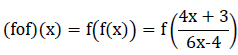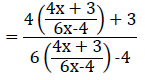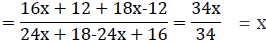⇒ fo f = Ix
Hence, the given function f is invertible and the inverse of f is f itself.

Q.5. State with reason whether following functions have inverse
(i) f : {1, 2, 3, 4} → {10} with f = {(1, 10), (2, 10), (3, 10), (4, 10)}
(ii) g: {5, 6, 7, 8} → {1, 2, 3, 4} with g = {(5, 4), (6, 3), (7, 4), (8, 2)}
(iii) h: {2, 3, 4, 5} → {7, 9, 11, 13} with h = {(2, 7), (3, 9), (4, 11), (5, 13)}

Ans.
(i) f: {1, 2, 3, 4} → {10} defined as f = {(1, 10), (2, 10), (3, 10), (4, 10)}
From the given definition of f, we can see that f is a many one function as
f(1) = f(2) = f(3) = f(4) = 10
∴ f is not one – one.
Hence, function f does not have an inverse.

(ii) g: {5, 6, 7, 8} → {1, 2, 3, 4} defined as
g = {(5, 4), (6, 3), (7, 4), (8, 2)}
From the given definition of g, it is seen that g is a many one function as
g(5) = g(7) = 4.
∴ g is not one – one.
Hence, function g does not have an inverse.

(iii) h: {2, 3, 4, 5} → {7, 9, 11, 13} defined as
h = {(2, 7), (3, 9), (4, 11), (5, 13)}
It is seen that all distinct elements of the set {2, 3, 4, 5} have distinct images under h.
∴ Function h is one – one.
Also, h is onto since for every element y of the set {7, 9, 11, 13}, there exists an element x in the set {2, 3, 4, 5}, such that h(x) = y.
Thus, h is a one – one and onto function.
Hence, h has an inverse.

Q.6. Show that f: [−1, 1] → R, given by f(x) = x/x + 2 is one – one. Find the inverse of the function f: [−1, 1] → Range f.
(Hint: For y ∈ Range f, y =
f(x) = x/x + 2), for some x in [−1, 1], i.e.,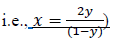Ans.
f : [−1, 1] → R is given as f(x) = x/x + 2
For one – one
Let f(x) = f(y)
⇒ x/x + 2 = y/y + 2
⇒ xy + 2x = xy + 2y
⇒ 2x = 2y
⇒ x = y
∴ f is a one – one function.
It is clear that f: [−1, 1] → Range f is onto.
∴ f: [−1, 1] → Range f is one – one and onto and therefore, the inverse of the function f: [−1, 1] → Range f exists.
Let g: Range f → [−1, 1] be the inverse of f.
Let y be an arbitrary element of range f.
Since f: [−1, 1] → Range f is onto, we have
y = f(x) for some x ∈ [−1, 1]
⇒ y = xx + 2
⇒ xy + 2y = x
⇒ x(1 - y) = 2y
⇒ x = 2y/1 - y, y≠1
Now, let us define g: Range f → [−1, 1] as
g(y) = 2y/1 - y, y ≠ 1
Now,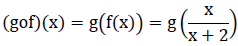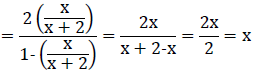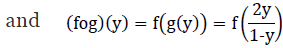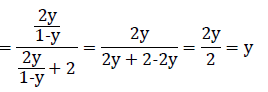∴ gof = x = I[-1, 1] and fog = y = IRange f
∴ f-1 = g
⇒ f-1(y) = 2y/1 - y, y ≠ 1

Q.7. Consider f: R → R given by f(x) = 4x + 3. Show that f is invertible. Find the inverse of f.
Ans.
f: R → R is given by, f(x) = 4x + 3
For one – one
Let f(x) = f(y)
⇒ 4x + 3 = 4y + 3
⇒ 4x = 4y
⇒ x = y
∴ f is a one – one function.
For onto
For y ∈ R, let y = 4x + 3.
⇒ x = y - 3/4 ∈ R
Therefore, for any y ∈ R, there exists x = y - 34 ∈ R, such that
f(x) = f(y - 3/4) = 4(y - 3/4) + 3 = y.
∴ f is onto.
Thus, f is one – one and onto and therefore, f −1 exists.
Let us define g: R → R by g(x) = y-3/4
Now, (gof)(x) = g(f(x)) = g(4x + 3) = (4x + 3) - 3/4 = 4x/4 = x
and (fog)(y) = f(g(y)) = f(y - 3/4) = 4(y - 3/4) + 3 = y - 3 + 3 = y
∴ gof = fog = IR
Hence, f is invertible and the inverse of f is given by f - 1(y) = g(y) = y - 34.

Q.8. Consider f: R+ → [4, ∞] given by f(x) = x2 + 4. Show that f is invertible with the inverse f−1 of given f by f−1(y) = √y − 4, where R+ is the set of all non-negative real numbers.
Ans.
f : R+ → [4, ∞] is given as f(x) = x2 + 4.
For one – one
Let f(x) = f(y)
⇒ x2+4 = y2+4
⇒ x2 = y2
⇒ x = y [x = y, ∈ R+]
∴ f is a one – one function.
For onto For y ∈ [4, ∞), let y = x2 + 4
⇒ x2 = y − 4 ≥ 0 [as y ≥ 4]
⇒ x = √y - 4 ≥ 0
Therefore, for any y ∈ [4, ∞], there exists x = √y - 4 ∈ R+, such that f(x) = f(√y - 4) = (√y - 4)2 + 4 = y - 4 + 4 = y
∴ f is onto.
Thus, f is one – one and onto and therefore, f −1 exists.
Let us define g: [4, ∞] → R+ by g(y) = √y - 4
Now, (gof)(x) = g(f(x)) = g(x+ 4) = √(x+ 4) - 4 = √x2 = x
and (fog)(y) = f(g(y)) = f(√y - 4) = (√y - 4)2 + 4 = y - 4 + 4 = y
∴ gof = fog = IR
Hence, f is invertible and the inverse of f is given by f - 1(y) = g(y) = √y − 4

Q.9. Consider f: R+ → [−5, ∞] given by f(x) = 9x+ 6x − 5. Show that f is invertible with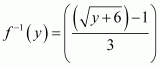.
Ans. f: R+ → [−5, ∞] is given as f(x) = 9x2 + 6x − 5.
Let y be an arbitrary element of [−5, ∞).
Let y  = 9x2 + 6x − 5.
⇒ y = (3x + 1)2 - 1 -5 = (3x + 1)2 - 6
⇒ (3x + 1)2 = y + 6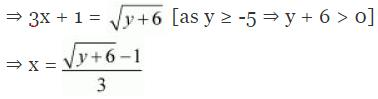∴ f is onto, thereby range f  = [−5, ∞).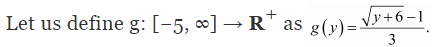We now have:
(gof) (x) = g (f(x)) = g(9x2 + 6x - 5)
= g ((3x + 1)2 - 6)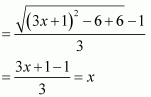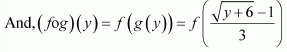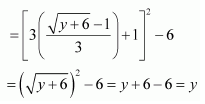∴ gof = IR. and fo g = I[-5, ∞)
Hence, f is invertible and the inverse of f is given by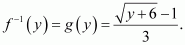Q.10. Let f: X → Y be an invertible function. Show that f has unique inverse.
(Hint: suppose g1 and g2 are two inverses of f. Then for all y ∈ Y, fog1(y) = IY(y) =  fog2(y). Use one-one ness of f).
Ans. Let f: X → Y be an invertible function.
Also, suppose f has two inverses (say g1 and g2).
Then, for all y ∈ Y, we have:
fog1(y) = Iy (y) = fog2 (y)
⇒ f(g1(y)) = f(g2(y))
⇒ g1(y) = g2(y) [ f is invertible ⇒ f is one-one]
⇒ g1 = g[g is one-one]
Hence, f has a unique inverse.

Q.11. Consider f: {1, 2, 3} → {a, b, c} given by f(1) = a, f(2) = b and f(3) =  c. Find f−1 and show that (f−1)−1  = f.
Ans. Function f: {1, 2, 3} → {a, b, c} is given by,
f(1) =  a, f(2) =  b, and f(3) =  c
If we define g: {a, b, c} → {1, 2, 3} as g(a) = 1, g(b) = 2, g(c) = 3, then we have:
(fog)(a) = f (g(a)) = f(1) = a
(fog)(b) = f(g(b)) = f(2) = b
(fog)(c) = f (g(c)) = f(3) = c
And,
(gof)(1) = g(f(1)) = g(a)=l
(gof)(2) = g(f(2)) = g(b) = 2
(gof) (3) = g (f (3)) = g(c) = 3
∴ gof = Ix and fog = Iy, where X = {1, 2, 3} and Y = {a, b, c}.
Thus, the inverse of f exists and f−1  =  g.
∴ f−1 : {a, b, c} → {1, 2, 3} is given by,
f−1(a) = 1, f−1(b) = 2, f-1(c) = 3
Let us now find the inverse of f−1 i.e., find the inverse of g.
If we define h: {1, 2, 3} → {a, b, c} as
h(1) =  a, h(2) =  b, h(3) =  c, then we have:
(goh)(1) = g(h(1)) = g(a) = 1
(goh)(2) = g(h(2)) = g(b) = 2
(goh) (3) = g (h(3)) = g(c) = 3
And,
(hog)(a) = h(g(a)) = h(1) = a
(hog)(b) = h(g(b)) = h(2) = b
(hog)(c) = h (g(c)) = h(3) = c
∴ goh = Ix and hog = Iy, where X  = {1, 2, 3} and Y = {a, b, c}.
Thus, the inverse of g exists and g−1 = h ⇒ (f−1)−1 = h.
It can be noted that h  =  f.
Hence, (f−1)−1  =  f.

Q.12. Let f: X → Y be an invertible function. Show that the inverse of f−1 is f, i.e., (f−1)−1  =  f.
Ans. Let f: X → Y be an invertible function.
Then, there exists a function g: Y → X such that gof  = Iand fog  = IY.
Here, f−1  =  g.
Now, gof  = Iand fog  = IY
⇒ f−1of  = Iand fof−1 = IY
Hence, f−1: Y → X is invertible and f is the inverse of f−1
i.e., (f−1)−1  =  f.

Q.13. If f : be given by f(x) = (3 - x3)1/3, then fof(x) is
(a) 1/x3
(b) x3
(c) x
(d) (3 − x3)

Ans. (c)
Solution.
f: RR is given as f(x) = (3 - x3)1/3.
f(x) = (3 - x3)1/3
∴ fof (x) = f (f(x)) = f((3 - x3)1/3) = [3 - ((3 - x3)1/3)3]1/3
= [3 - (3 - x3)]1/3 = (x3)1/3 = x
∴ fof (x) = x

Q.14.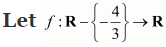be a function defined as f(x) = 4x/(3x+4). The inverse of f is map g: Range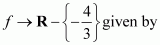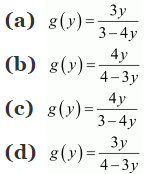Ans. (b)
Solution.
It is given that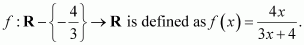Let y be an arbitrary element of Range f.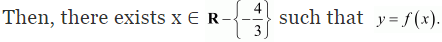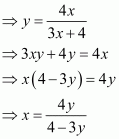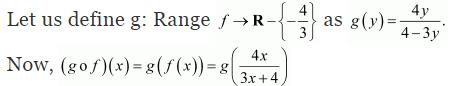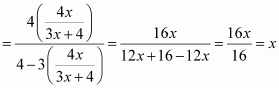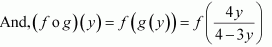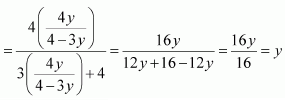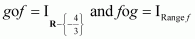Thus, g is the inverse of f i.e., f−1  =  g.
Hence, the inverse of f is the map g: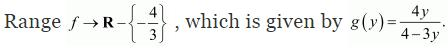Offer running on EduRev: Apply code STAYHOME200 to get INR 200 off on our premium plan EduRev Infinity!

## Mathematics (Maths) Class 12

209 videos|222 docs|124 tests

,

,

,

,

,

,

,

,

,

,

,

,

,

,

,

,

,

,

,

,

,

;